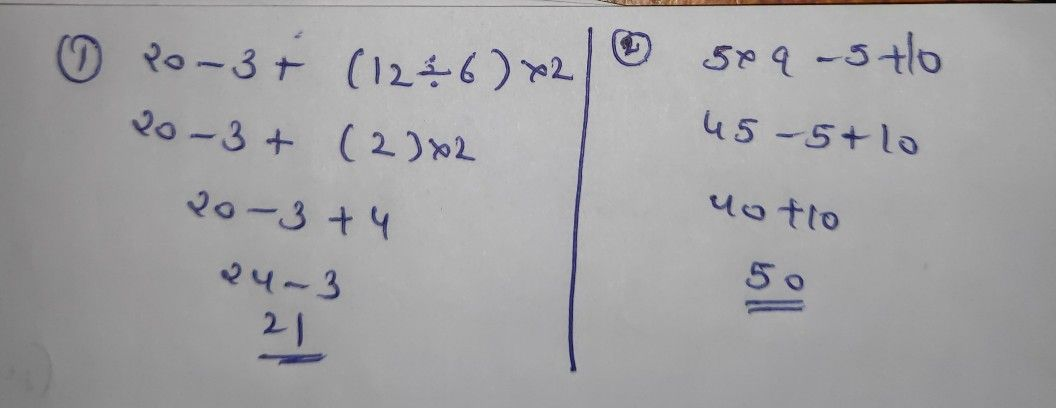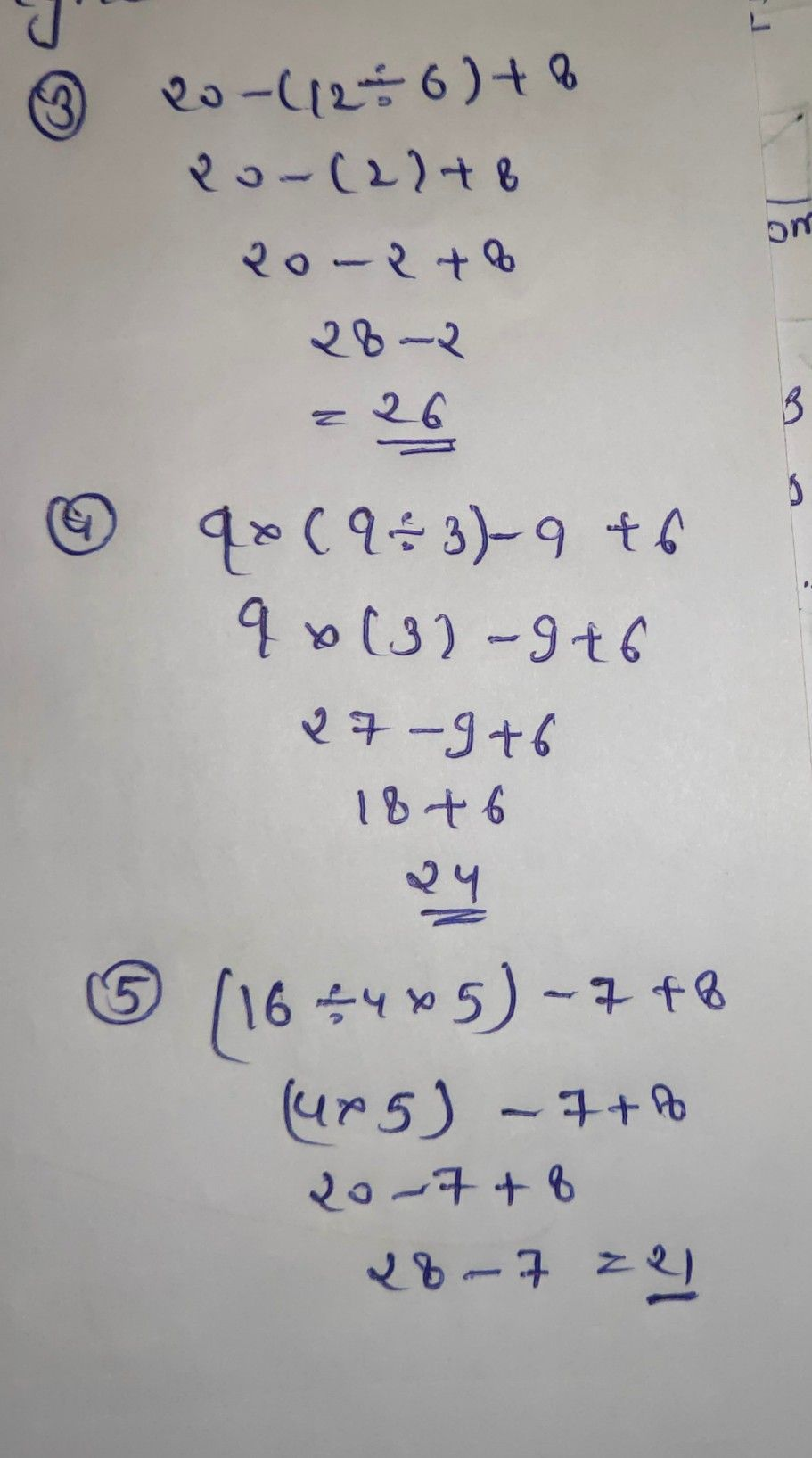Symbol
Problemcarning Task 1: Perform the indicated operations. Write your annwer in aveun notebook. $1\right)\left(12+3\right)-7=N$ $4\right)\left(18-4\right)+\left(5+3\right)=y$ $2.\right)4\left(6+8\right)=N$ $5.\right)\left(6+3\right)+\left(10\times 3\right)=N$ $3.\right)25+5+9=N$ Learning Task $23$ Perform the indicated operations $1Q$ solve the $\left(olox-ing$ Write your answer in your notebook. $1.20-3+\left(12\div 6\right)\times 2=$ $5\times 9-5+10=$ $2.5$ $3.20-\left(12\div 6\right)+8=$ $4.9\times \left(9+3\right)-9+6=$ $5.\left(16\div 4\times 5\right)-7+8=$ Learning Task $3:$ Perform the indicated operations to solve the $to1-lo$ $ng$ by following the $PMDAS$ and GMDAS rules. Write your answer in your notebook. $6.\right)4\times \left(15-13\right)+16=$
1st-6th grade
Calculus
Search count: 111
Question content
hi sirim grade5 student can u pls help me about my math prpblem thanks
SolutionQanda teacher - AskhinaThanks for asking a question. You can ask any doubt without any hesitation in a direct way also for high-quality answers quickly and please rate 5 stars if you are satisfied with my answers And, if you have no doubt related to the previous question then please evaluate the answer and give 3000 coins as a gift ( these are not reduced to your wallet)